# Consider the following time series data. Week 1 2 3 45 6 Value 20 12 15...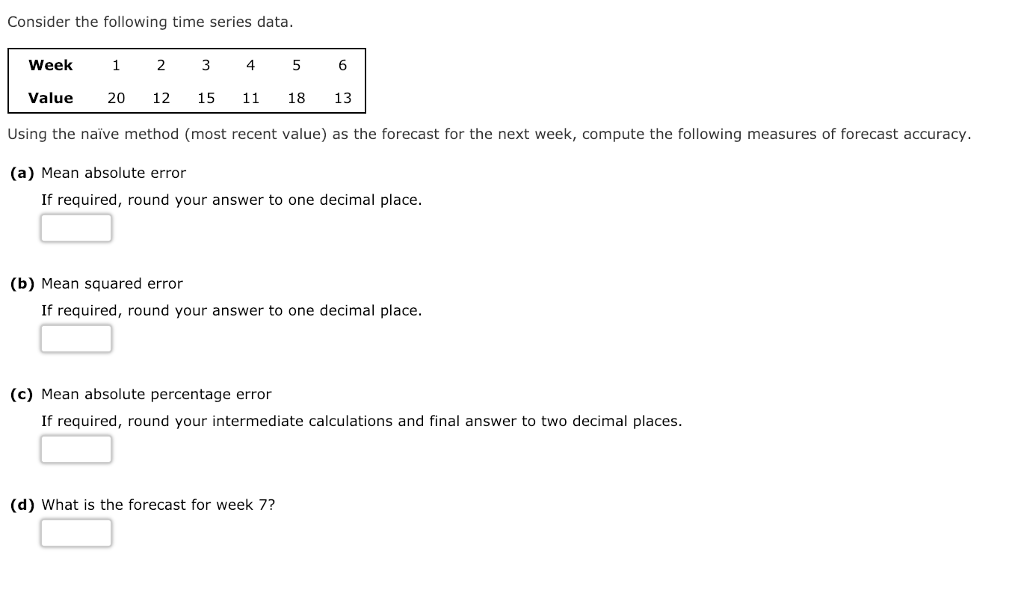Consider the following time series data. Week 1 2 3 45 6 Value 20 12 15 11 18 13 Using the naive method (most recent value) as the forecast for the next week, compute the following measures of forecast accuracy. (a) Mean absolute error If required, round your answer to one decimal place. (b) Mean squared error If required, round your answer to one decimal place. (c) Mean absolute percentage error If required, round your intermediate calculations and final answer to two decimal places. (d) What is the forecast for week 7?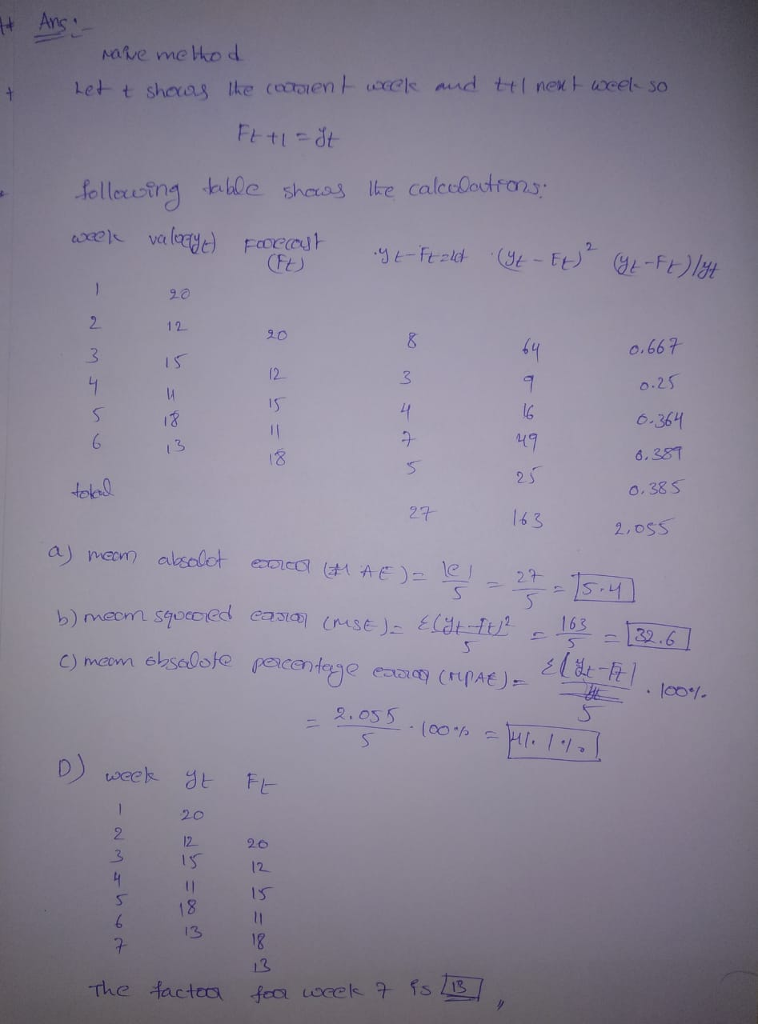##### Add Answer of: Consider the following time series data. Week 1 2 3 45 6 Value 20 12 15...
Similar Homework Help Questions
• ### Consider the following time series data. Week 1 2 3 45 6 Value 19 12 15...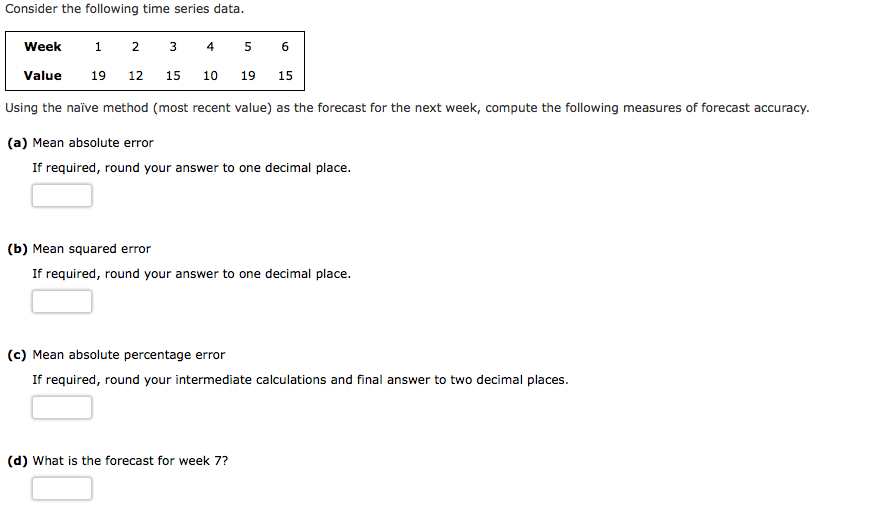Consider the following time series data. Week 1 2 3 45 6 Value 19 12 15 10 19 15 Using the naïve method (most recent value) as the forecast for the next week, compute the following measures of forecast accuracy. (a) Mean absolute error If required, round your answer to one decimal place. (b) Mean squared error If required, round your answer to one decimal place. (c) Mean absolute percentage error If required, round your intermediate calculations and final answer...

• ### the answer is not 41.1 OR -13.42 Consider the following time series data. Week12 345 6...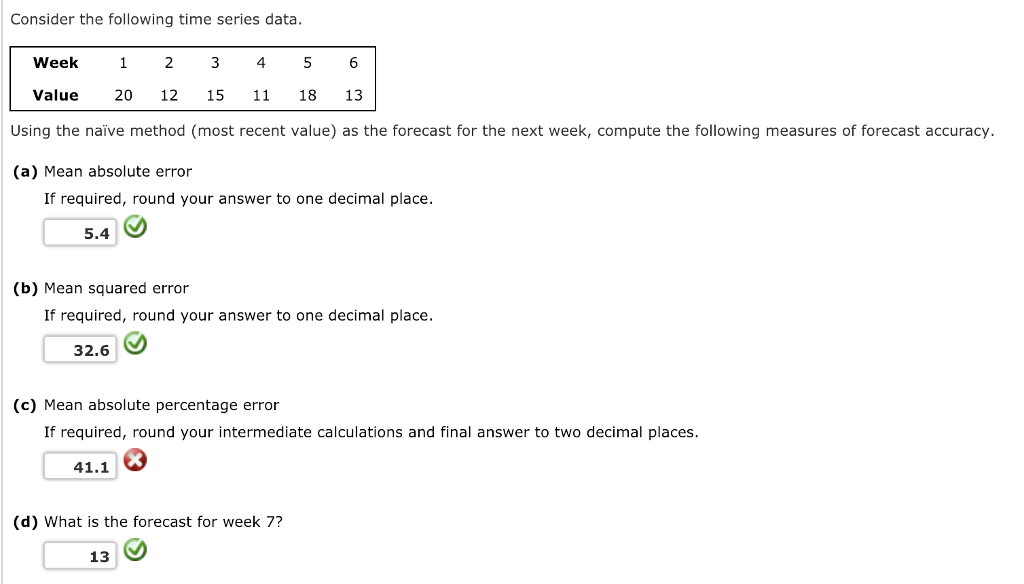the answer is not 41.1 OR -13.42 Consider the following time series data. Week12 345 6 Value 20 12 15 11 18 13 Using the naive method (most recent value) as the forecast for the next week, compute the following measures of forecast accuracy (a) Mean absolute error If required, round your answer to one decimal place 5.4 (b) Mean squared error If required, round your answer to one decimal place. 32.6 (c) Mean absolute percentage error If required, round...

• ### Problem 15-01 (Algorithmic) Consider the following time series data. Week 1 2 3 4 6 Value...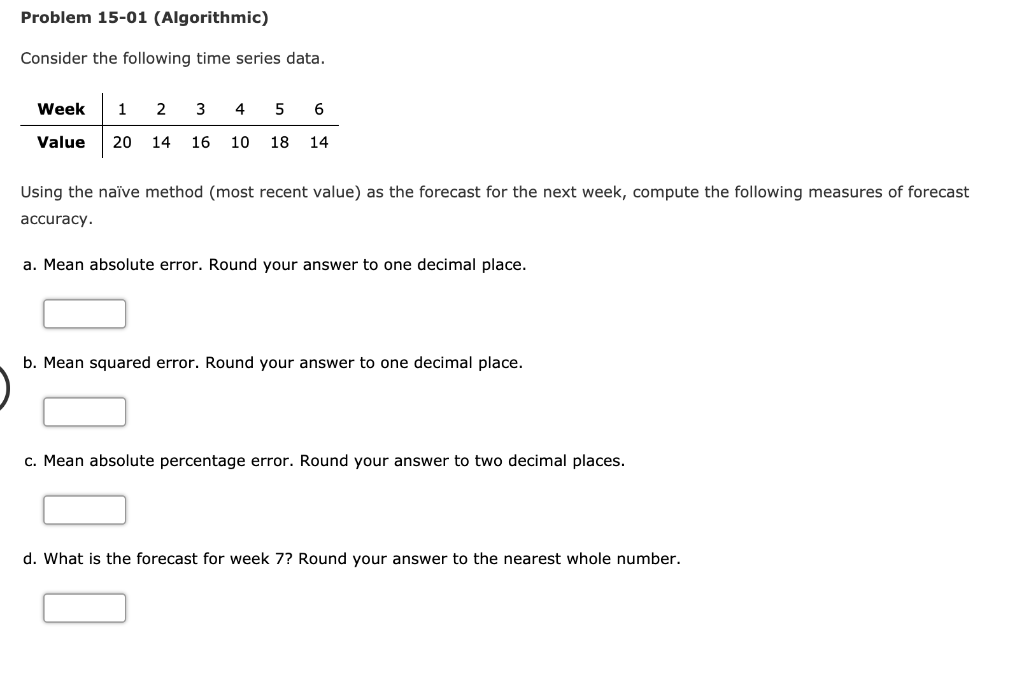Problem 15-01 (Algorithmic) Consider the following time series data. Week 1 2 3 4 6 Value 20 14 16 10 18 14 Using the naiïve method (most recent value) as the forecast for the next week, compute the following measures of forecast accuracy a. Mean absolute error. Round your answer to one decimal place. b. Mean squared error. Round your answer to one decimal place. c. Mean absolute percent error. Round you answer to two decimal places. d. What is...

• ### Consider the following time series data. Week 1 2 3 4 5 6 Value 1914 16...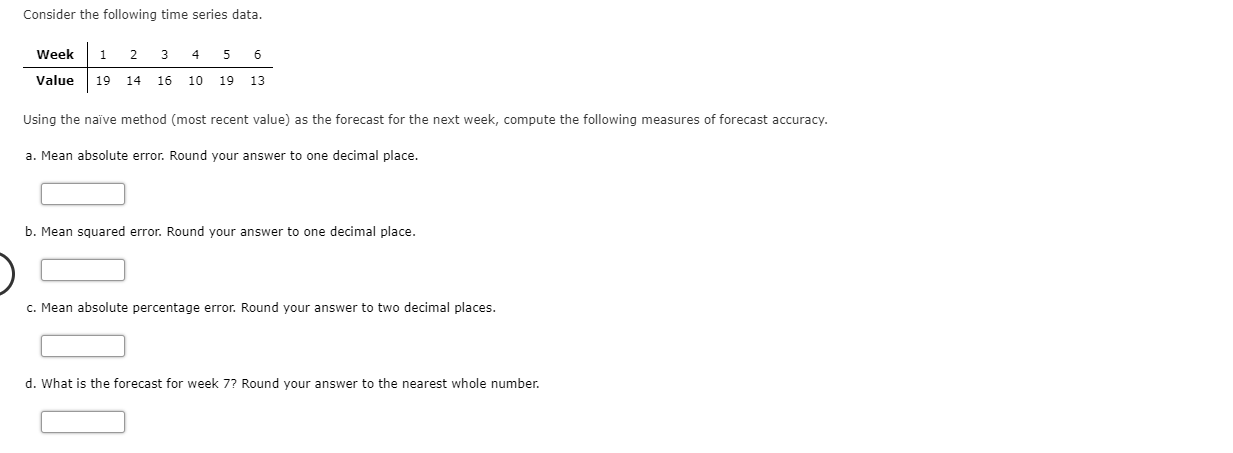Consider the following time series data. Week 1 2 3 4 5 6 Value 1914 16 10 19 13 Using the naïve method (most recent value) as the forecast for the next week, compute the following measures of forecast accuracy. a. Mean absolute error. Round your answer to one decimal place. b. Mean squared error. Round your answer to one decimal place. c. Mean absolute percentage error. Round your answer to two decimal places. d. What is the forecast for...

• ### Consider the following time series data. Week 1 2 3 4 5 6 Value 18 13...

Consider the following time series data. Week 1 2 3 4 5 6 Value 18 13 17 11 17 15 Calculate the measures of forecast error using the naive (most recent value) method and the average of historical data (to 2 decimals). Naive method Historical data Mean absolute error Mean squared error Mean absolute percentage error Top of Form Consider the following time series data. Month 1     2 3 4 5 6 7 Value 23 14 19 10 18 23...

• ### Consider the following time series data. Week 1 2 3 4 5 6 Value 20 13...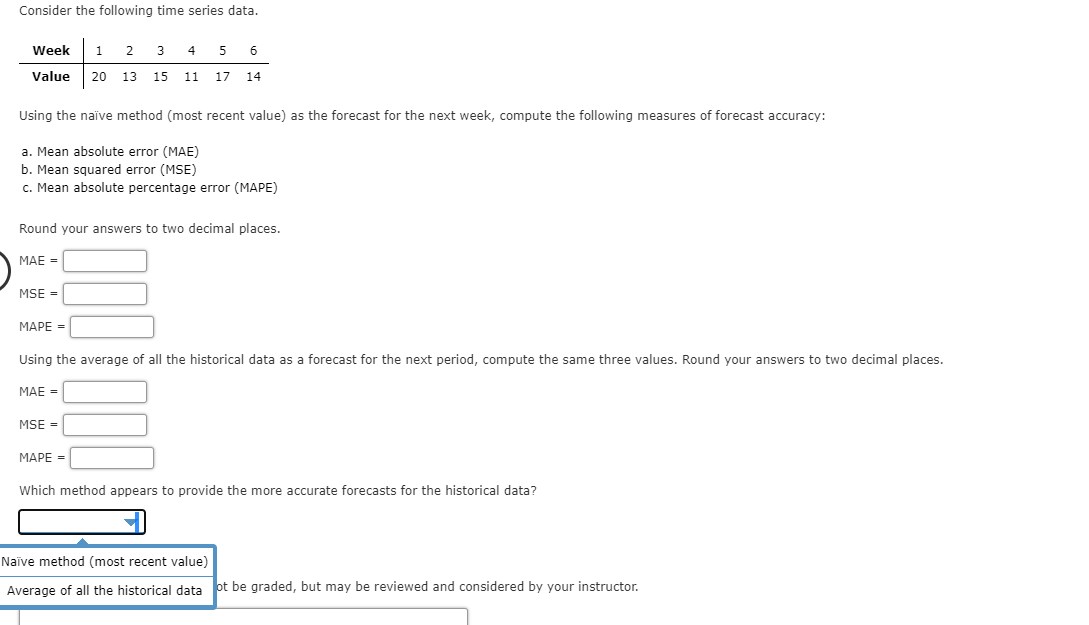Consider the following time series data. Week 1 2 3 4 5 6 Value 20 13 15 11 17 14 Using the naïve method (most recent value) as the forecast for the next week, compute the following measures of forecast accuracy: a. Mean absolute error (MAE) b. Mean squared error (MSE) c. Mean absolute percentage error (MAPE) Round your answers to two decimal places. MAE = MSE = MAPE = Using the average of all the historical data as a...

• ### Please help Consider the following time series data. 3 4 5 6 Week 12 18 14...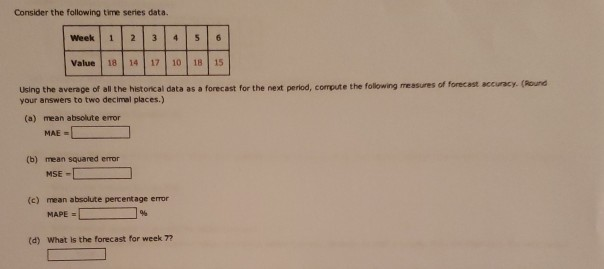Please help Consider the following time series data. 3 4 5 6 Week 12 18 14 Value 17 1018 15 Using the average of all the historical data as a forecast for the next period, compute the following measures of forecast accuracy. (Round your answers to two decimal places.) (a) mean absolute error MAE - (b) mean squared error MSE - (c) mean absolute percentage error MAPE 96 (d) What is the forecast for week 7?

• ### Consider the following time series data: Month Value 1 1 25 2 12 3 20 4...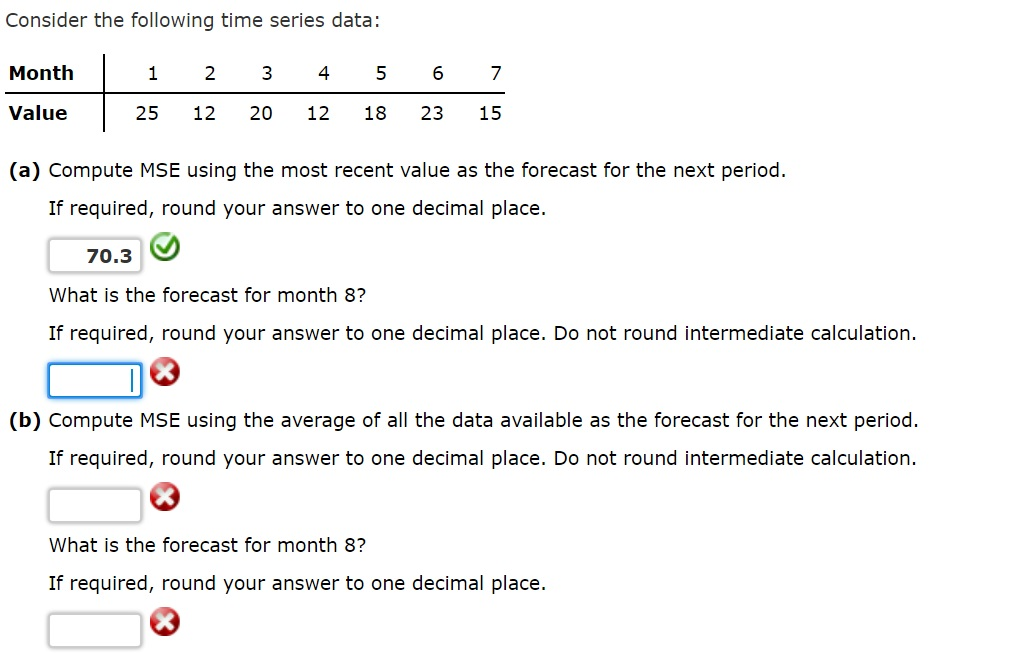Consider the following time series data: Month Value 1 1 25 2 12 3 20 4 12 5 18 6 23 7 15 (a) Compute MSE using the most recent value as the forecast for the next period. If required, round your answer to one decimal place. 70.3 What is the forecast for month 8? If required, round your answer to one decimal place. Do not round intermediate calculation. 1 (b) Compute MSE using the average of all the data...

• ### Consider the following time series data. Week 1 2 3 4 5 6 Value 18 13...

Consider the following time series data. Week 1 2 3 4 5 6 Value 18 13 16 11 17 14 (a) Choose the correct time series plot. (i) (ii) (iii) (iv) - Select your answer -Plot (i)Plot (ii)Plot (iii)Plot (iv)Item 1 What type of pattern exists in the data? - Select your answer -Horizontal PatternTrend PatternItem 2 (b) Develop a three-week moving average for this time series. Compute MSE and a forecast for week 7. Do not round intermediate calculations....

• ### PLEASE ONLY ANSWER IF YOU CAN DO WHAT IS BLANK, I DON'T NEED HELP WITH WHAT...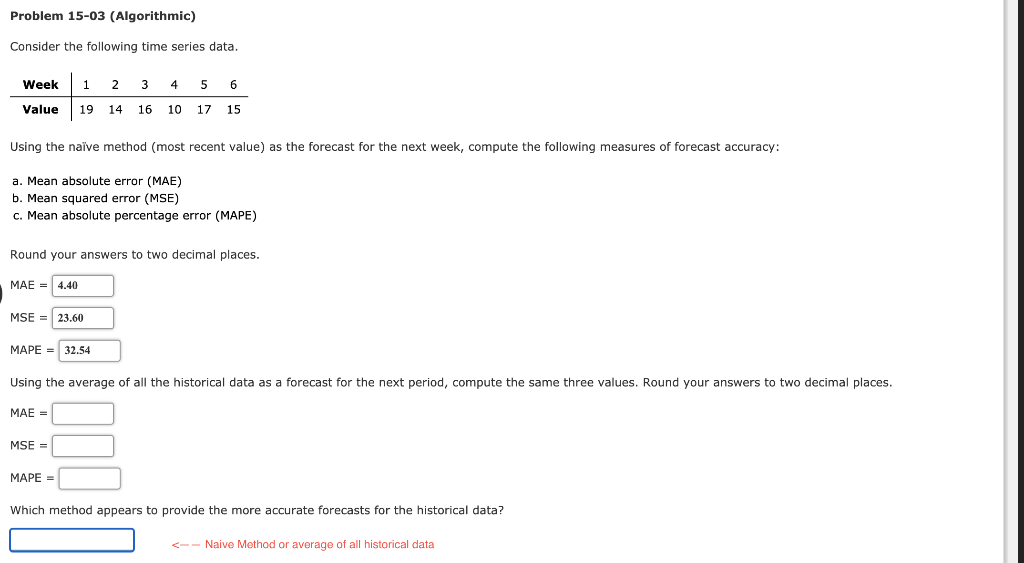PLEASE ONLY ANSWER IF YOU CAN DO WHAT IS BLANK, I DON'T NEED HELP WITH WHAT IS FILLED OUT ALREADY. THANK YOU! Problem 15-03 (Algorithmic) Consider the following time series data. Week 1 2 3 4 5 6 Value 1914 16 10 17 15 Using the naive method (most recent value) as the forecast for the next week, compute the following measures of forecast accuracy: a. Mean absolute error (MAE) b. Mean squared error (MSE) c. Mean absolute percentage error...

Free Homework App